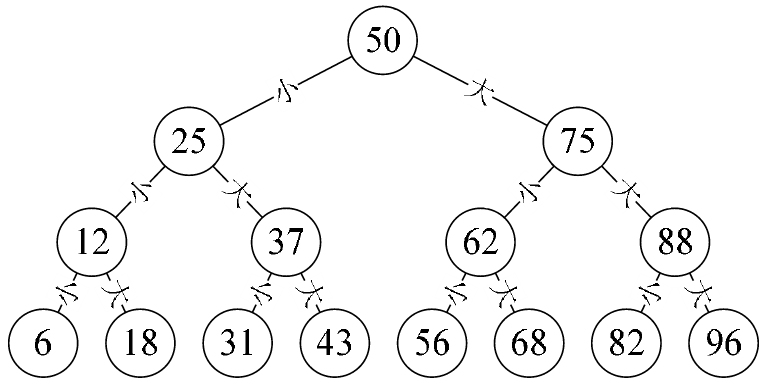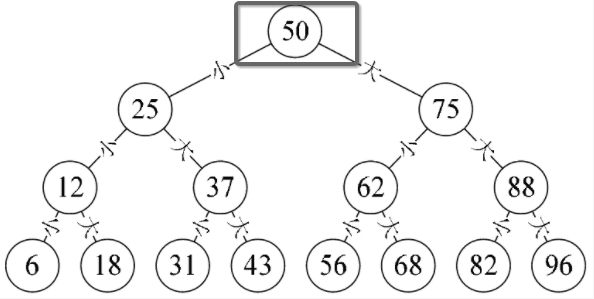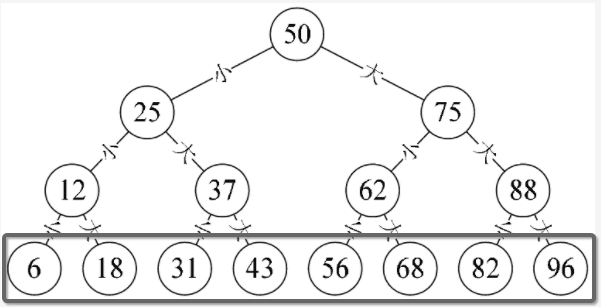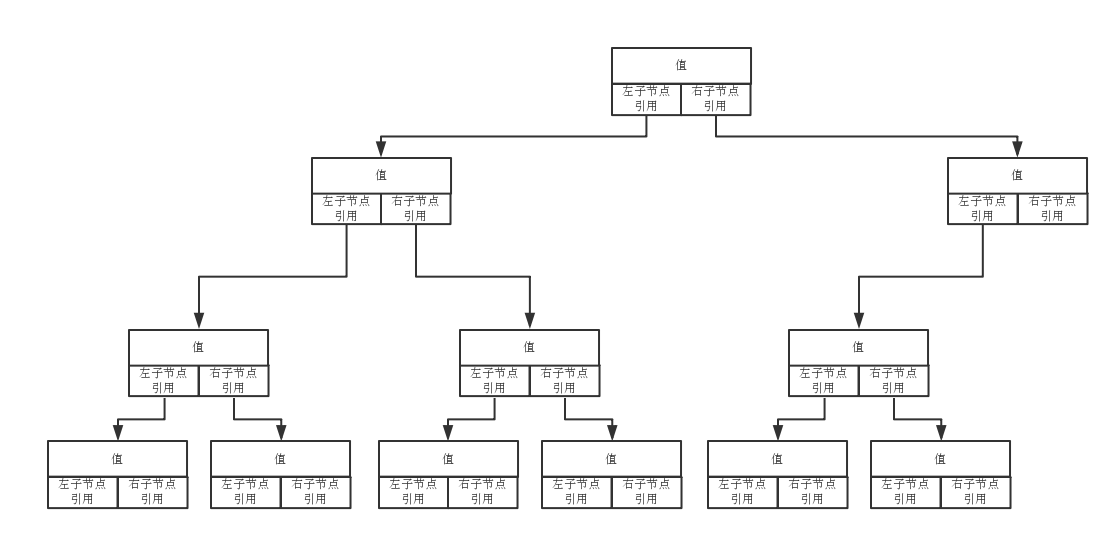# javascript实现二叉树的代码

更新时间：2017年06月08日 11:25:57   作者：issac_宝华我要评论1. 除了最下面一层，每个节点都是父节点，每个节点都有且最多有两个子节点；
2. 除了嘴上面一层，每个节点是子节点，每个节点都会有一个父节点；
3. 最上面一层的节点（即例图中的节点50）为根节点；1 节点的javascript实现

```// 节点对象
function Node(data, left, right) {
this.data = data; // 节点值
this.left = left; // 当前节点的左子节点
this.right = right; // 当前节点的右子节点
this.show = show; // 辅助function
}

function show() {
return this.data;
}
```2 二叉树的实现

```function BST() {
this.root = null;
this.insert = insert;
}

function insert(data) {
var n = new Node(data, null, null);
if (this.root == null) {
this.root = n;
}
else {
var current = this.root;
var parent;
while (true) {
parent = current;
if (data < current.data) {
current = current.left;
if (current == null) {
parent.left = n;
break;
}
}
else {
current = current.right;
if (current == null) {
parent.right = n;
break;
}
}
}
}
}

```

```function BST() {
this.root = null; // 根节点
this.insert = insert;
}

function insert(data) {
// 初始化一个节点，为什么要将左右子节点的引用初始化为空呢，因为可能是叶子节点，加入他有子节点，会在下面的代码添加
var n = new Node(data, null, null);
if (该二叉树是否为空，是空则根节点为空，因此可以用根节点判断二叉树是否为空) {
// 将当前节点存为根节点
this.root = n;
}
else {
// 来到这里就表示，该二叉树不为空，这里关键的是两句代码：
// 0.while (true);
// 1.parent = current；
// 2.current = current.left;/current = current.right;
// 3.break;
var current = this.root;
var parent;
while (true) {
parent = current; // 获得父节点，第一次循环，那么父节点就是根节点
if (data < current.data) { // 当前节点值小于父节点的值就是存左边，记得二叉树的特点吧，如果真是小于父节点，那么就说明该节点属于，该父节点的左子树。
current = current.left;
if (current == null) {
parent.left = n;
break;
}

// 其实上面这样写不好理解，可以等价于下面的代码：
// start
if(current.left == null){ // 若果左节点空，那么这个空的节点就是我们要插入的位置
current.left = n;
break;
}else{
// 不空则继续往下一层找空节点（插入的位置）
current = current.left;
}
// end
}
else {
// 右节点的逻辑代码个左节点的一样的
current = current.right;
if (current == null) {
parent.right = n;
break;
}
}
}
}
}

```

```function insert(data) {
var n = new Node(data, null, null);
if (this.root == null) {
this.root = n;
}
else {
var current = this.root;
// start change
while (true) {
if (data < current.data) {
if (current.left == null) {
current.left = n;
break;
}else{
current = current.left;
}
}else {
if (current.right == null) {
current.right = n;
break;
}else{
current = current.right;
}
}
}
}
}
```

Node对象

`function Node(data, left, right) { ... }`

BST对象

`function BST() { ... }`

`function insert(data) { ... }`